k-means_k-means算法 - CSDN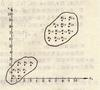k-means 订阅
k均值聚类算法（k-means clustering algorithm）是一种迭代求解的聚类分析算法，其步骤是，预将数据分为K组，则随机选取K个对象作为初始的聚类中心，然后计算每个对象与各个种子聚类中心之间的距离，把每个对象分配给距离它最近的聚类中心。聚类中心以及分配给它们的对象就代表一个聚类。每分配一个样本，聚类的聚类中心会根据聚类中现有的对象被重新计算。这个过程将不断重复直到满足某个终止条件。终止条件可以是没有（或最小数目）对象被重新分配给不同的聚类，没有（或最小数目）聚类中心再发生变化，误差平方和局部最小。 展开全文k均值聚类算法（k-means clustering algorithm）是一种迭代求解的聚类分析算法，其步骤是，预将数据分为K组，则随机选取K个对象作为初始的聚类中心，然后计算每个对象与各个种子聚类中心之间的距离，把每个对象分配给距离它最近的聚类中心。聚类中心以及分配给它们的对象就代表一个聚类。每分配一个样本，聚类的聚类中心会根据聚类中现有的对象被重新计算。这个过程将不断重复直到满足某个终止条件。终止条件可以是没有（或最小数目）对象被重新分配给不同的聚类，没有（或最小数目）聚类中心再发生变化，误差平方和局部最小。

1967年

James MacQueen

K均值聚类算法

k-means clustering algorithm
K均值聚类算法定义

• K-means原理,python实现，改进，sklearn应用，SPSS应用。所谓物以类聚，人以群分。相似的人们总是相互吸引在一起。数据也是一样。在kNN中，某个数据以与其他数据间的相似度来预测其标签，而K-means是一群无标记数据...
K-means原理：
所谓物以类聚，人以群分。相似的人们总是相互吸引在一起。数据也是一样。在kNN中，某个数据以与其他数据间的相似度来预测其标签，而K-means是一群无标记数据间的因为自我相似的聚拢。显而易见，K-means的目标为簇内密集而簇间稀疏。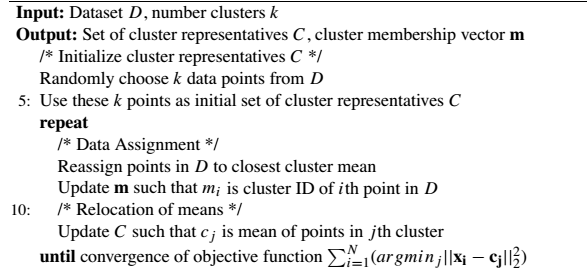简单来说就是首先先确定k个初始点作为质心，然后将数据集中的每一个点分配到一个距其最近的簇中，这一步完成后将每个簇的质心更新为该簇所有点的平均值，直至中心不再变化。
算法实现：（Python）
def distEclud(vecA, vecB):#计算两个向量的欧式距离
return sqrt(sum(power(vecA - vecB, 2)))

def randCent(dataSet, k):#随机质心
n = shape(dataSet)
centroids = mat(zeros((k,n)))
for j in range(n):
minJ = min(dataSet[:,j])
rangeJ = float(max(dataSet[:,j]) - minJ)
centroids[:,j] = mat(minJ + rangeJ * random.rand(k,1))
return centroids

def kMeans(dataSet, k, distMeas=distEclud, createCent=randCent):
m = shape(dataSet)
clusterAssment = mat(zeros((m,2)))#存储每个点的分配，即簇索引值和误差
centroids = createCent(dataSet, k)
clusterChanged = True
while clusterChanged:
clusterChanged = False
for i in range(m):
minDist = inf; minIndex = -1
for j in range(k):#寻找最近质心
distJI = distMeas(centroids[j,:],dataSet[i,:])
if distJI < minDist:
minDist = distJI; minIndex = j
if clusterAssment[i,0] != minIndex: clusterChanged = True
clusterAssment[i,:] = minIndex,minDist**2
print (centroids)
for cent in range(k):#更新质心的位置
ptsInClust = dataSet[nonzero(clusterAssment[:,0].A==cent)]
centroids[cent,:] = mean(ptsInClust, axis=0)
return centroids, clusterAssment

k-means算法简单、快速，效率高，当数据集是密集的、球状或团状的簇群，且簇与簇之间区别明显时，聚类效果很好。但是它也有不少的问题：
使用范围的约束
k-means，k-means，这个平均值（means）被定义的情况下才能使用。而且对有些分类属性的数据不适用。而且对非凸面形状的簇，或者大小差别很大的簇简直无解。
k是用户指派的？k的选择问题
由于k是用户预先定义的参数，那么如何选择k才是最佳的呢？一种度量聚类效果的指标是误差平方和（Sum of Squared Error，SSE），SSE越小表示数据点越接近它们的质心，聚类的效果相对来说也就最好。所以利用SEE的改进方法就是对生成的簇进行后处理，一是将最大的SSE值的簇分成两个（将这个簇的点过滤出后再进行k为2的k-means），而是合并最小的SSE簇。
或者是人工考虑“肘部法则”来选择，即画出不同k值下的代价函数图，这很像一个人的肘部，观察图形可知，当达到一个点时J下降的非常快，之后会很慢，由此来选择k。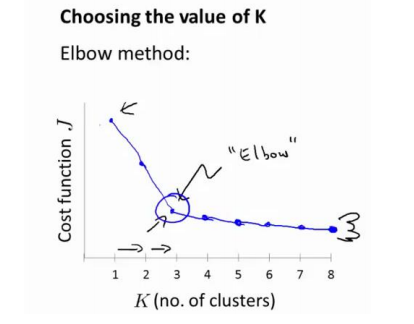但是当遇到高维度、海量的数据集时，人们往往很难准确地估计出K的大小，所以最好使用迭代自组织数据分析法（Iterative Self-Organizing Data Analysis Technique ，ISODATA）来判断。即当属于某个类别的样本数过少时把这个类别去除，当属于某个类别的样本数过多、分散程度较大时把这个类别分为两个子类别。
只能用欧式距离？度量方式的选择
除了根据实际情况进行选择外，还可以参照支持向量机中核函数的思想，将所有样本映射到另外一个特征空间中再进行聚类。
闵可夫斯基距离
欧几里得距离
曼哈顿距离
切比雪夫距离
马氏距离
余弦相似度
皮尔逊相关系数
汉明距离
杰卡德相似系数
编辑距离
DTW 距离
KL 散度
计算距离时间太多？距离度量的优化
elkan K-Means算法利用了两边之和大于等于第三边,以及两边之差小于第三边的三角形性质，来减少计算量，提高时间。
不抗干扰，被噪声扰动影响太大
初质心不同，结果不同？K-means对初值的敏感
K-means非常容易受初识质心的影响，质心的不同，聚类结果可能千差万别。所以为了更好的效果，可以采用二分k均值聚类，即每次都选择有最大误差的簇进行二分，直至k个簇全部创建成功。
算法实现：（Python）
def biKmeans(dataSet, k, distMeas=distEclud):#二分k均值聚类
m = shape(dataSet)
clusterAssment = mat(zeros((m,2)))
centroid0 = mean(dataSet, axis=0).tolist()
centList =[centroid0]
for j in range(m):
clusterAssment[j,1] = distMeas(mat(centroid0), dataSet[j,:])**2
while (len(centList) < k):
lowestSSE = inf
for i in range(len(centList)):#遍历每一个簇
ptsInCurrCluster = dataSet[nonzero(clusterAssment[:,0].A==i),:]
centroidMat, splitClustAss = kMeans(ptsInCurrCluster, 2, distMeas)
sseSplit = sum(splitClustAss[:,1])#计算划分后的两个簇的误差
sseNotSplit = sum(clusterAssment[nonzero(clusterAssment[:,0].A!=i),1])
print ("sseSplit, and notSplit: ",sseSplit,sseNotSplit)
if (sseSplit + sseNotSplit) < lowestSSE:#误差之和作为本次划分的误差
bestCentToSplit = i
bestNewCents = centroidMat
bestClustAss = splitClustAss.copy()
lowestSSE = sseSplit + sseNotSplit
bestClustAss[nonzero(bestClustAss[:,0].A == 1),0] = len(centList) #更新簇的分配结果。将刚刚划分完的0，1编号的结果簇修改编号，由两个数组过滤器来完成
bestClustAss[nonzero(bestClustAss[:,0].A == 0),0] = bestCentToSplit
print ('the bestCentToSplit is: ',bestCentToSplit)
print ('the len of bestClustAss is: ', len(bestClustAss))
centList[bestCentToSplit] = bestNewCents[0,:].tolist()#新的质心增加到centroids中
centList.append(bestNewCents[1,:].tolist())
clusterAssment[nonzero(clusterAssment[:,0].A == bestCentToSplit),:]= bestClustAss
return mat(centList), clusterAssment

原型聚类
K-means之所以为原型聚类就在于它是通过先对原型进行初始化，然后对原型进行不断的迭代求解。当然了，不同的原型刻画方法，求解方式，将产生不同的算法。对K-means来说，初始的k个样本便是它的原型向量。与K-means一样，学习向量量化（Learning Vector Quantization，LVQ）也是尝试找到一组原型向量来刻画该原型，但LVQ的样本是带有标记的，即通过监督信息来辅助聚类。如果你了解一些神经网络，那么LVQ对于你应该很熟悉，它的核心其实就是“适者生存”的竞争策略，只对竞争获胜的神经元进行参数调整，是自组织映射(Self-organizing Maps,SOM)基于监督信息的一种变体。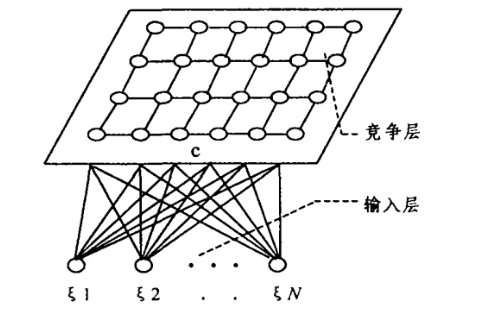而它用于聚类也是如此。大致算法思想是从样本集中随机选取一个有标记的样本（x，y），找到最小的那个原型向量p，判断样本的标记y与原型向量的标记是否一致。若一致则更新为p’ = p + a*(x-p)，否则更新为p’ = p - a*(x - p)。 直观上看标记相同则靠拢，不同则远离。
K-means应用：
KMeans参数说明：KMeans(algorithm=‘auto’, copy_x=True, init=‘k-means++’, max_iter=300,n_clusters=3, n_init=10, n_jobs=1, precompute_distances=‘auto’,random_state=None, tol=0.0001, verbose=0)
algorithm："full"就是传统的K-Means算法， “elkan”是采用elkan K-Means算法。
copy_x=True：对是否修改数据的一个标记，如果True，即复制了就不会修改数据。
init='k-means++'：初始值选择方式
max_iter=300：最大迭代
n_clusters=3：k的值
n_init=10：初始化质心运行次数
n_jobs=1：并行工作数
precompute_distances='auto'：是否预计算距离
random_state=None：随机状态条件
tol=0.0001： 容忍度，即kmeans运行准则收敛的条件
verbose=0：冗长模式

MiniBatchKMeans(batch_size=45, compute_labels=True, init=‘k-means++’, init_size=None, max_iter=100, max_no_improvement=10, n_clusters=3,n_init=10, random_state=None, reassignment_ratio=0.01, tol=0.0,verbose=0)
batch_size=45：采样集大小
compute_labels=True：计算标签
init='k-means++'：初始值选择方式
init_size=None：质心选择的样本数
max_iter=100：最大迭代
max_no_improvement=10：连续性的无改善聚类效果的最大阈值
n_clusters=3：k的值
n_init=10：初始化质心运行次数
random_state=None：随机状态条件
reassignment_ratio=0.01：某个类别质心被重新赋值的最大次数比例
tol=0.0：容忍度，即kmeans运行准则收敛的条件
verbose=0：冗长模式

比较这两种模式的聚类表现：
import time

import numpy as np
import matplotlib.pyplot as plt

from sklearn.cluster import MiniBatchKMeans, KMeans
from sklearn.metrics.pairwise import pairwise_distances_argmin
from sklearn.datasets.samples_generator import make_blobs

#s生成数据
np.random.seed(0)

batch_size = 45
centers = [[1, 1], [-1, -1], [1, -1]]
n_clusters = len(centers)
X, labels_true = make_blobs(n_samples=3000, centers=centers, cluster_std=0.7)

#Means
k_means = KMeans(init='k-means++', n_clusters=3, n_init=10)
t0 = time.time()
k_means.fit(X)
t_batch = time.time() - t0

#MiniBatchKMeans
mbk = MiniBatchKMeans(init='k-means++', n_clusters=3, batch_size=batch_size,
n_init=10, max_no_improvement=10, verbose=0)
t0 = time.time()
mbk.fit(X)
t_mini_batch = time.time() - t0

fig=plt.figure(figsize=(8, 3))
colors = ['#4EACC5', '#FF9C34', '#4E9A06']

k_means_cluster_centers = np.sort(k_means.cluster_centers_, axis=0)
mbk_means_cluster_centers = np.sort(mbk.cluster_centers_, axis=0)
k_means_labels = pairwise_distances_argmin(X, k_means_cluster_centers)
mbk_means_labels = pairwise_distances_argmin(X, mbk_means_cluster_centers)
order = pairwise_distances_argmin(k_means_cluster_centers,
mbk_means_cluster_centers)

# KMeans
for k, col in zip(range(n_clusters), colors):
my_members = k_means_labels == k
cluster_center = k_means_cluster_centers[k]
ax.plot(X[my_members, 0], X[my_members, 1], 'w',
markerfacecolor=col, marker='.')
ax.plot(cluster_center, cluster_center, 'o', markerfacecolor=col,
markeredgecolor='k', markersize=6)
ax.set_title('KMeans')
ax.set_xticks(())
ax.set_yticks(())
plt.text(-3.5, 1.8,  'train time: %.2fs\ninertia: %f' % (
t_batch, k_means.inertia_))

# MiniBatchKMeans
for k, col in zip(range(n_clusters), colors):
my_members = mbk_means_labels == order[k]
cluster_center = mbk_means_cluster_centers[order[k]]
ax.plot(X[my_members, 0], X[my_members, 1], 'w',
markerfacecolor=col, marker='.')
ax.plot(cluster_center, cluster_center, 'o', markerfacecolor=col,
markeredgecolor='k', markersize=6)
ax.set_title('MiniBatchKMeans')
ax.set_xticks(())
ax.set_yticks(())
plt.text(-3.5, 1.8, 'train time: %.2fs\ninertia: %f' %
(t_mini_batch, mbk.inertia_))

#两者的不同聚类点
different = (mbk_means_labels == 4)

for k in range(n_clusters):
different += ((k_means_labels == k) != (mbk_means_labels == order[k]))

identic = np.logical_not(different)
ax.plot(X[identic, 0], X[identic, 1], 'w',
markerfacecolor='#bbbbbb', marker='.')
ax.plot(X[different, 0], X[different, 1], 'w',
markerfacecolor='m', marker='.')
ax.set_title('Difference')
ax.set_xticks(())
ax.set_yticks(())

plt.show()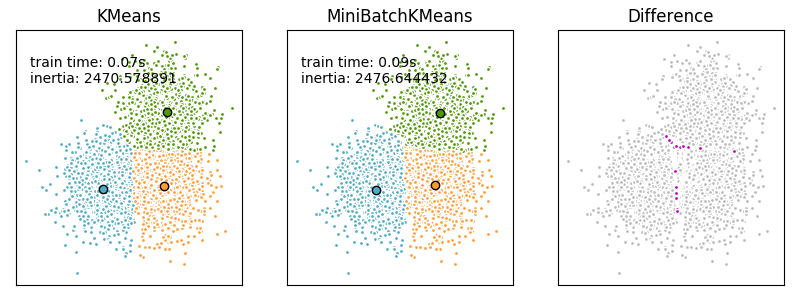可以发现两者聚类结果只有少量的不同。
SPSS应用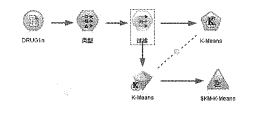详细介绍就不多说了，运行后打开节点可以看到很多的细节。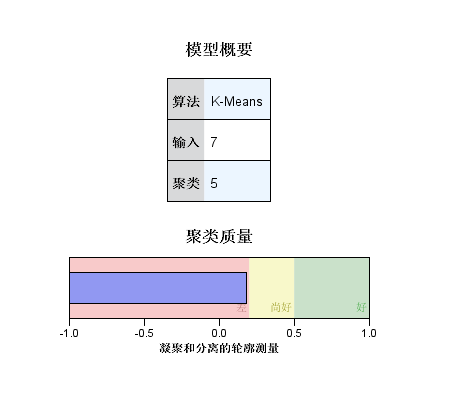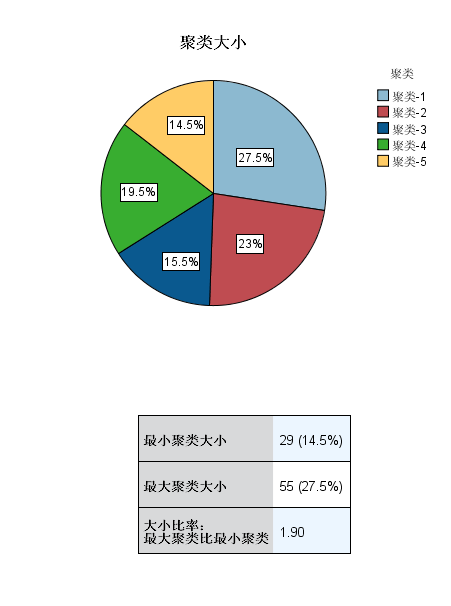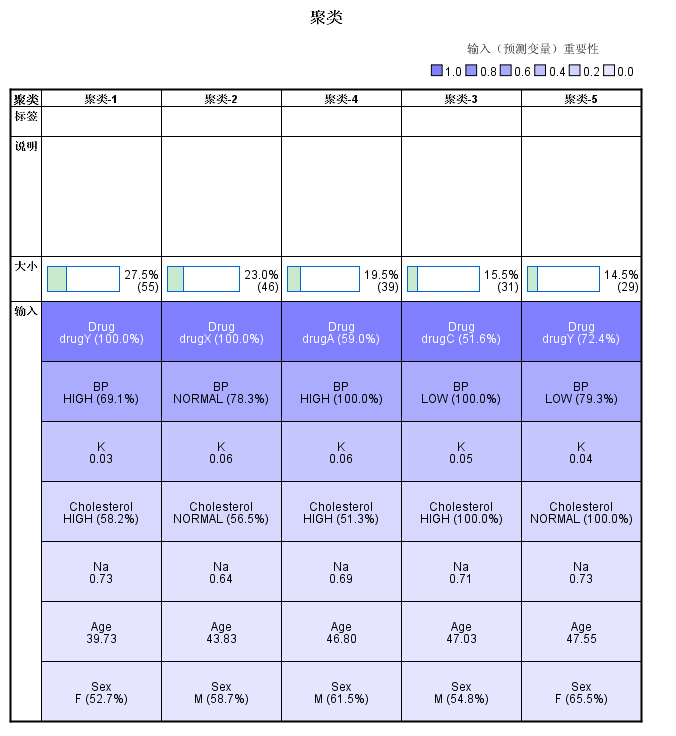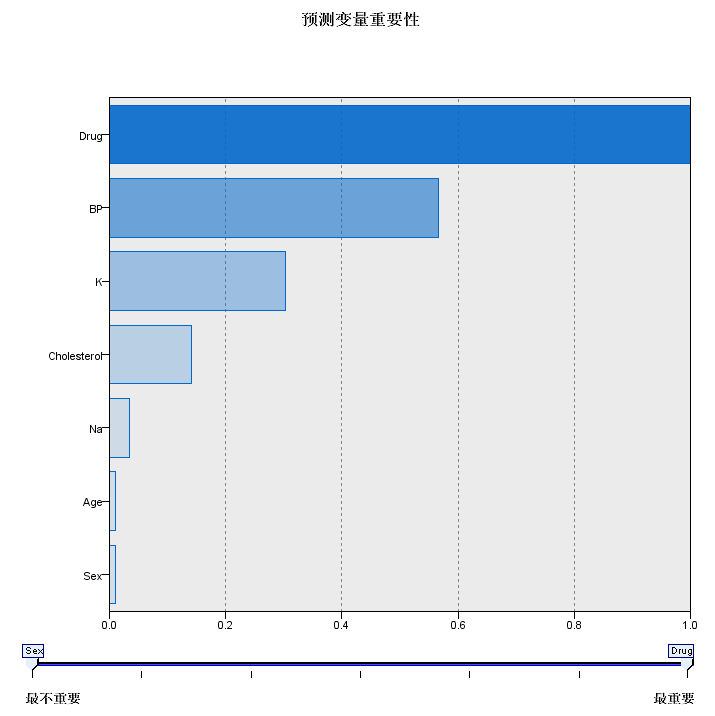和Weka一样，画好图后应用就可以得到结果：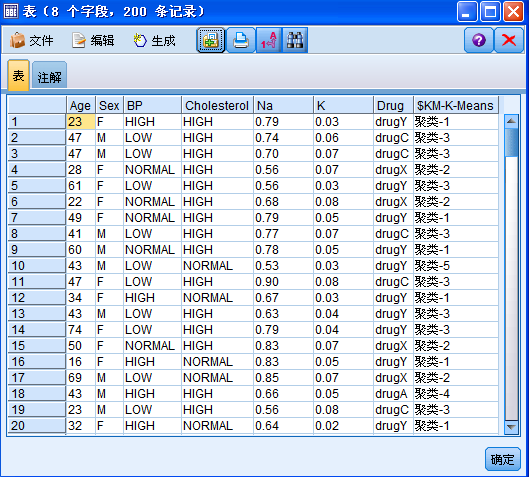展开全文k-means python weka 聚类
• K-means算法的主要思想就是以空间中的K个点为中心进行聚类，对最靠近它的对象进行归类。通过迭代的方法不断的更新各聚类中心的值，直到最好的聚类结果。 主要步骤： 在N个数据中，随机挑选K个数据（也就是最后...
K-means算法的主要思想就是以空间中的K个点为中心进行聚类，对最靠近它的对象进行归类。通过迭代的方法不断的更新各聚类中心的值，直到最好的聚类结果。

K的取值：
确定聚类数K没有最佳的方法，通常需要根据具体的问题由人工进行选择。非监督聚类没有比较直接的聚类评估方法，但是可以从簇内的稠密程度和簇间的离散程度来评估聚类的效果。最常见的方法有轮廓系数Silhouette Coefficient和Calinski-Harabaz Index。其中Calinski-Harabaz Index计算直接简单，得到的结果越大则聚类效果越好。计算公式如下：
$s(K)=(\frac{tr(B_{k})}{tr(W_{k})}\cdot \frac{m-k}{k-1})$
其中：m为训练集样本数，k为类别数。Bk为类别之间的协方差矩阵，Wk为内部数据之间的协方差矩阵。tr为矩阵的迹。
也就是说内部数据的协方差越小越好，类别之间的协方差越大越好，这样对应的Calinski-Harabaz Index分数也就越高

主要步骤：

在N个数据中，随机挑选K个数据（也就是最后聚类为K类）做为聚类的初始中心。
分别计算每个数据点到这K个中心点的欧式距离，离哪个中心点最近就分配到哪个簇中。
重新计算这K个簇数据的坐标均值，将新的均值作为聚类的中心。
重复2和3步骤，直到簇中心的坐标不再变换或者达到规定的迭代次数，形成最终的K个聚类。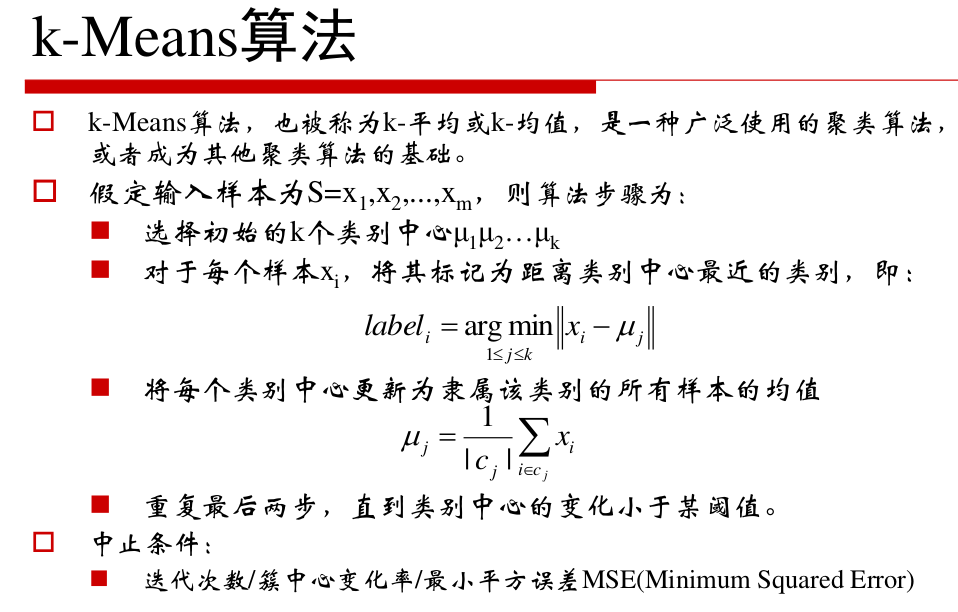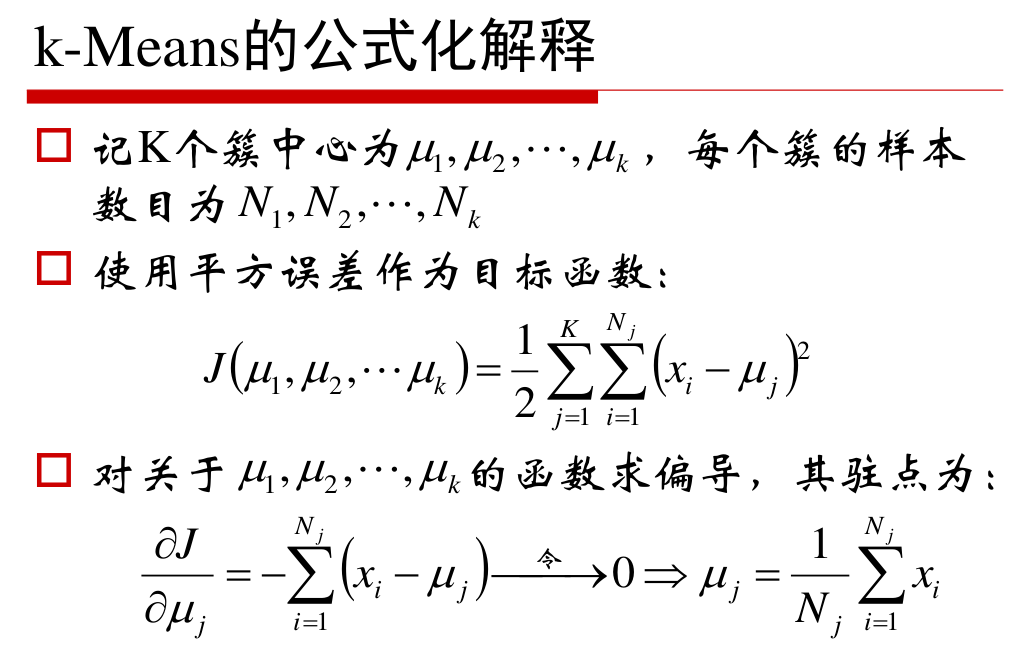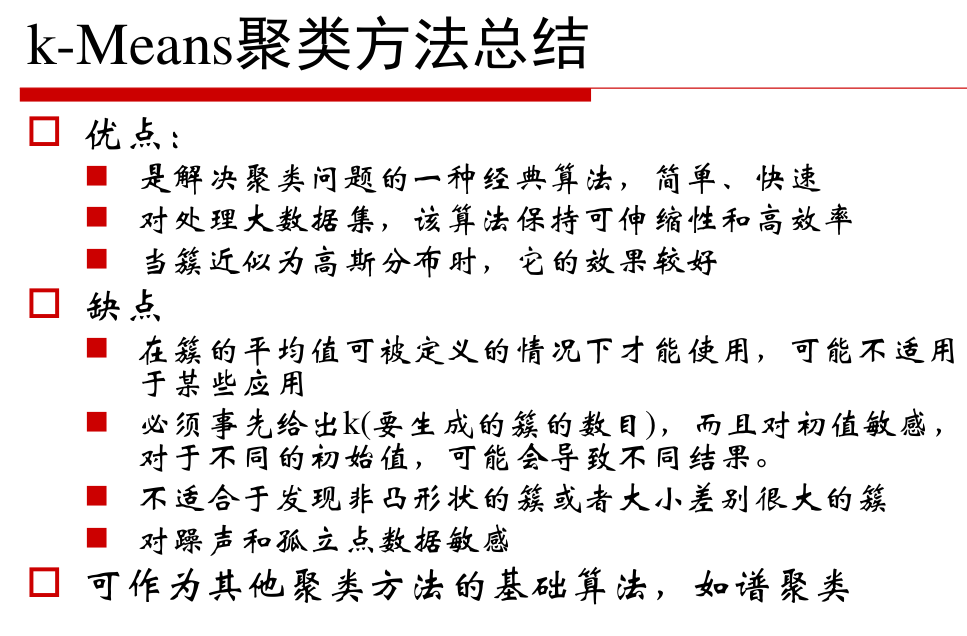源码实现：
有两个py文件。kmeans.py 和 kmeanstest.py。源码在kmeans.py中，kmeanstest.py为实例（后期会给出sklean运行代码）。
# %load kmeans.py

#导入模块
import numpy as np
import matplotlib.pyplot as plt
from math import sqrt

#计算欧式距离
def eucDistance(vec1,vec2):
return sqrt(sum(pow(vec2-vec1,2)))

#初始聚类中心选择
def initCentroids(dataSet,k):
numSamples,dim = dataSet.shape
centroids = np.zeros((k,dim))
for i in range(k):
index = int(np.random.uniform(0,numSamples))
centroids[i,:] = dataSet[index,:]
return centroids

#K-means聚类算法，迭代
def kmeanss(dataSet,k):
numSamples = dataSet.shape
clusterAssement = np.mat(np.zeros((numSamples,2)))
clusterChanged = True
#  初始化聚类中心
centroids = initCentroids(dataSet,k)
while clusterChanged:
clusterChanged = False
for i in range(numSamples):
minDist = 100000.0
minIndex = 0
# 找到哪个与哪个中心最近
for j in range(k):
distance = eucDistance(centroids[j,:],dataSet[i,:])
if distance<minDist:
minDist = distance
minIndex = j
# 更新簇
clusterAssement[i,:] = minIndex,minDist**2
if clusterAssement[i,0]!=minIndex:
clusterChanged = True
# 坐标均值更新簇中心
for j in range(k):
pointsInCluster = dataSet[np.nonzero(clusterAssement[:0].A==j)]
centroids[j,:] = np.mean(pointsInCluster,axis=0)
print('Congratulations,cluster complete!')
return centroids,clusterAssement

#聚类结果显示
def showCluster(dataSet,k,centroids,clusterAssement):
numSamples,dim = dataSet.shape
mark = ['or','ob','og','ok','^r','+r','<r','pr']
if k>len(mark):
print('Sorry!')
return 1
for i in np.xrange(numSamples):
markIndex = int(clusterAssement[i,0])
plt.plot(centroids[i,0],centroids[i,1],mark[i],markersize=12)
plt.show()

有如下80个二维数据的样本，存在testSet的文档中，经过数据预处理和分析得知数据集共有4个类，所以确定K=4。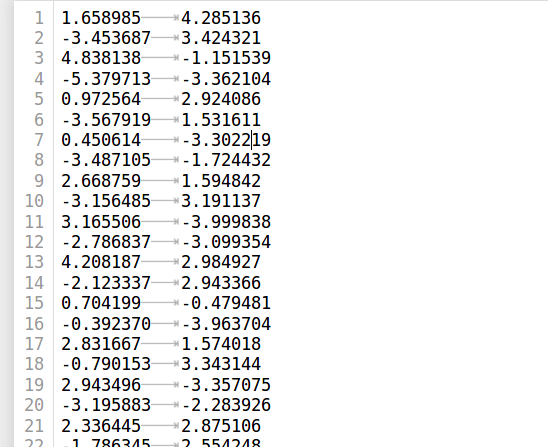# %load kmeanstest.py

#导入模块
import kmeans
import  numpy as np
import matplotlib.pyplot as plt
from math import sqrt

#从文件加载数据集
dataSet=[]
fileIn = open('./testSet.txt')
lineArr = line.strip().split('\t')
dataSet.append([float(lineArr),float(lineArr)])

#调用k-means进行数据聚类
dataSet = np.mat(dataSet)
k = 4
centroids,clusterAssement = kmeans.kmeanss(dataSet,k)

#显示结果
kmeans.showCluster(dataSet,centroids,clusterAssement)

使用k-means算法聚类结果如下：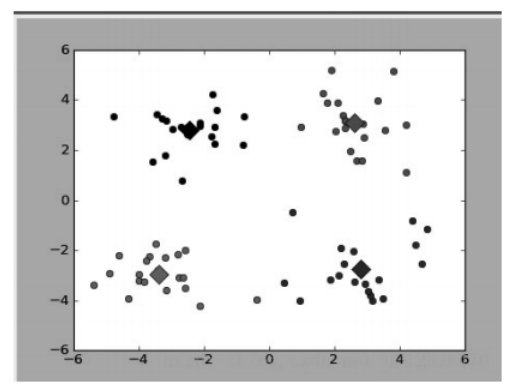用sklearn实现Kmeans：
随机生成二维多类数据，样本大致分为4类，调用sklearn中的聚类函数进行分析不同k值对聚类结果的影响以及评估方法。
import numpy as np
import matplotlib.pyplot as plt
from sklearn.datasets.samples_generator import make_blobs
from sklearn.cluster import KMeans

X, y = make_blobs(n_samples=1000, n_features=2, centers=[[-1,-1], [0,0], [1,1], [2,2]],\
cluster_std = [0.4, 0.2, 0.2, 0.2], random_state=9)
plt.scatter(X[:, 0], X[:, 1], marker='+')
plt.show()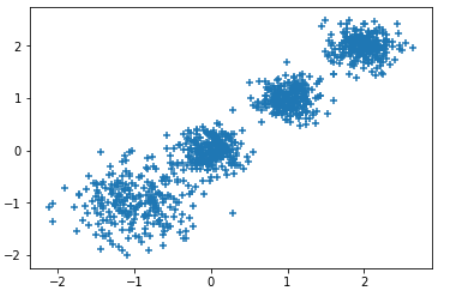K=2：
y_pred = KMeans(n_clusters=2, random_state=9).fit_predict(X)
plt.scatter(X[:, 0], X[:, 1], c=y_pred)
plt.show()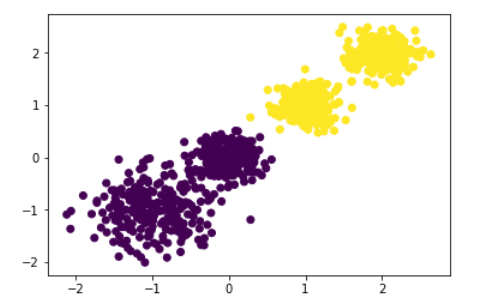调用Calinski-Harabaz Index来评估聚类分数:
from sklearn import metrics
metrics.calinski_harabaz_score(X, y_pred)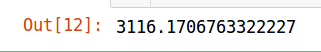K=3:
y_pred = KMeans(n_clusters=3, random_state=9).fit_predict(X)
plt.scatter(X[:, 0], X[:, 1], c=y_pred)
plt.show()
from sklearn import metrics
metrics.calinski_harabaz_score(X, y_pred)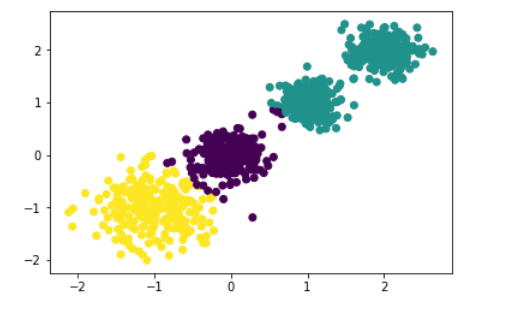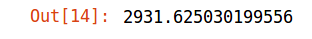K=4:
y_pred = KMeans(n_clusters=4, random_state=9).fit_predict(X)
plt.scatter(X[:, 0], X[:, 1], c=y_pred)
plt.show()
from sklearn import metrics
metrics.calinski_harabaz_score(X, y_pred)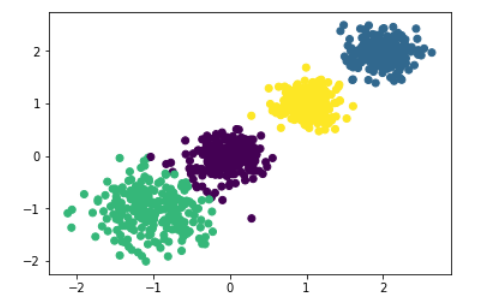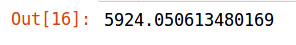可以发现当K=4的时候得分最高，在往后分值就会有所下降，所以现在的K值比较合适。
K=5:
y_pred = KMeans(n_clusters=5, random_state=9).fit_predict(X)
plt.scatter(X[:, 0], X[:, 1], c=y_pred)
plt.show()
from sklearn import metrics
metrics.calinski_harabaz_score(X, y_pred)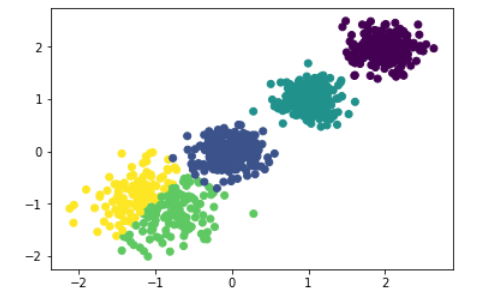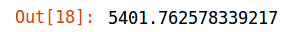展开全文kmeans 机器学习 聚类
• ## K-means原理、优化及应用

万次阅读 多人点赞 2018-08-23 14:48:59
K-Means算法有大量的变体，本文就从最传统的K-Means算法讲起，在其基础上讲述K-Means的优化变体方法。包括初始化优化K-Means++, 距离计算优化elkan K-Means算法和大数据情况下的优化Mini Batch K-Means算法。 1. K-...
　K-Means算法是无监督的聚类算法，它实现起来比较简单，聚类效果也不错，因此应用很广泛。K-Means算法有大量的变体，本文就从最传统的K-Means算法讲起，在其基础上讲述K-Means的优化变体方法。包括初始化优化K-Means++, 距离计算优化elkan K-Means算法和大数据情况下的优化Mini Batch K-Means算法。

1. K-Means原理初探

K-Means算法的思想很简单，对于给定的样本集，按照样本之间的距离大小，将样本集划分为K个簇。让簇内的点尽量紧密的连在一起，而让簇间的距离尽量的大。

如果用数据表达式表示，假设簇划分为(C1,C2,...Ck)(C1,C2,...Ck)，则我们的目标是最小化平方误差E：

E=∑i=1k∑x∈Ci||x−μi||22E=∑i=1k∑x∈Ci||x−μi||22

其中μiμi是簇CiCi的均值向量，有时也称为质心，表达式为：

μi=1|Ci|∑x∈Cixμi=1|Ci|∑x∈Cix

如果我们想直接求上式的最小值并不容易，这是一个NP难的问题，因此只能采用启发式的迭代方法。

K-Means采用的启发式方式很简单，用下面一组图就可以形象的描述。上图a表达了初始的数据集，假设k=2。在图b中，我们随机选择了两个k类所对应的类别质心，即图中的红色质心和蓝色质心，然后分别求样本中所有点到这两个质心的距离，并标记每个样本的类别为和该样本距离最小的质心的类别，如图c所示，经过计算样本和红色质心和蓝色质心的距离，我们得到了所有样本点的第一轮迭代后的类别。此时我们对我们当前标记为红色和蓝色的点分别求其新的质心，如图4所示，新的红色质心和蓝色质心的位置已经发生了变动。图e和图f重复了我们在图c和图d的过程，即将所有点的类别标记为距离最近的质心的类别并求新的质心。最终我们得到的两个类别如图f。

当然在实际K-Mean算法中，我们一般会多次运行图c和图d，才能达到最终的比较优的类别。

2. 传统K-Means算法流程

算法步骤：

1.（随机）选择K个聚类的初始中心；

2.对任意一个样本点，求其到K个聚类中心的距离，将样本点归类到距离最小的中心的聚类，如此迭代n次；

3.每次迭代过程中，利用均值等方法更新各个聚类的中心点(质心)；

4.对K个聚类中心，利用2,3步迭代更新后，如果位置点变化很小(可以设置阈值)，则认为达到稳定状态，迭代结束，对不同的聚类块和聚类中心可选择不同的颜色标注。

优点
1）原理比较简单，实现也是很容易，收敛速度快。
2）聚类效果较优。
3）算法的可解释度比较强。
4）主要需要调参的参数仅仅是簇数k。

缺点
1）K值的选取不好把握
2）对于不是凸的数据集比较难收敛
3）如果各隐含类别的数据不平衡，比如各隐含类别的数据量严重失衡，或者各隐含类别的方差不同，则聚类效果不佳。
4） 最终结果和初始点的选择有关，容易陷入局部最优。
5） 对噪音和异常点比较的敏感。

3. K-Means初始化优化K-Means++

k个初始化的质心的位置选择对最后的聚类结果和运行时间都有很大的影响，因此需要选择合适的k个质心。如果仅仅是完全随机的选择，有可能导致算法收敛很慢。K-Means++算法就是对K-Means随机初始化质心的方法的优化。

K-Means++的对于初始化质心的优化策略也很简单，如下：

a)  从输入的数据点集合中随机选择一个点作为第一个聚类中心μ1
b) 对于数据集中的每一个点xi，计算它与已选择的聚类中心中最近聚类中心的距离D(xi)=argmin||xi−μr||^2……r=1,2,...kselected

c) 选择下一个新的数据点作为新的聚类中心，选择的原则是：D(x)较大的点，被选取作为聚类中心的概率较大
d) 重复b和c直到选择出k个聚类质心
e) 利用这k个质心来作为初始化质心去运行标准的K-Means算法

K-Means++对初始聚类中心点的选取做了优化，简要来说就是使初始聚类中心点尽可能的分散开来，这样可以有效的减少迭代次数，加快运算速度。

4. K-Means距离计算优化elkan K-Means

在传统的K-Means算法中，我们在每轮迭代时，要计算所有的样本点到所有的质心的距离，这样会比较的耗时。那么，对于距离的计算有没有能够简化的地方呢？elkan K-Means算法就是从这块入手加以改进。它的目标是减少不必要的距离的计算。那么哪些距离不需要计算呢？

elkan K-Means利用了两边之和大于等于第三边,以及两边之差小于第三边的三角形性质，来减少距离的计算。

第一种规律是对于一个样本点xx和两个质心μj1,μj2。如果我们预先计算出了这两个质心之间的距离D(j1,j2)，则如果计算发现2D(x,j1)≤D(j1,j2),我们立即就可以知道D(x,j1)≤D(x,j2)。此时我们不需要再计算D(x,j2),也就是说省了一步距离计算。

第二种规律是对于一个样本点x和两个质心μj1,μj2。我们可以得到D(x,j2)≥max{0,D(x,j1)−D(j1,j2)}。这个从三角形的性质也很容易得到。

利用上边的两个规律，elkan K-Means比起传统的K-Means迭代速度有很大的提高。但是如果我们的样本的特征是稀疏的，有缺失值的话，这个方法就不使用了，此时某些距离无法计算，则不能使用该算法。

个人理解，第一个规律简单有效，很容易理解，但是第二个规律对简化运算量的意义不是很大。

5. 大样本优化Mini Batch K-Means

在统的K-Means算法中，要计算所有的样本点到所有的质心的距离。如果样本量非常大，比如达到10万以上，特征有100以上，此时用传统的K-Means算法非常的耗时，就算加上elkan K-Means优化也依旧。在大数据时代，这样的场景越来越多。此时Mini Batch K-Means应运而生。

顾名思义，Mini Batch，也就是用样本集中的一部分的样本来做传统的K-Means，这样可以避免样本量太大时的计算难题，算法收敛速度大大加快。当然此时的代价就是我们的聚类的精确度也会有一些降低。一般来说这个降低的幅度在可以接受的范围之内。

在Mini Batch K-Means中，我们会选择一个合适的批样本大小batch size，我们仅仅用batch size个样本来做K-Means聚类。那么这batch size个样本怎么来的？一般是通过无放回的随机采样得到的。

为了增加算法的准确性，我们一般会多跑几次Mini Batch K-Means算法，用得到不同的随机采样集来得到聚类簇，选择其中最优的聚类簇。

该算法的迭代步骤有两步：

1：从数据集中随机抽取一些数据形成小批量，把他们分配给最近的质心

2：更新质心

与K均值算法相比，数据的更新是在每一个小的样本集上。对于每一个小批量，通过计算平均值得到更新质心，并把小批量里的数据分配给该质心，随着迭代次数的增加，这些质心的变化是逐渐减小的，直到质心稳定或者达到指定的迭代次数，停止计算

Mini Batch K-Means比K-Means有更快的 收敛速度，但同时也降低了聚类的效果，但是在实际项目中却表现得不明显。个人理解，Mini Batch K-Means通过小样本的实验，可以初步预测聚类中心的位置，对n次Mini Batch K-Means的聚类结果取均值作为大样本实验初始聚类中心的位置，也能够减少运算量，加快聚类速度。

下边给出显示上边这副图的代码，也是对K-Means和Mini Batch K-Means算法的一个比较：

import time

import numpy as np
import matplotlib.pyplot as plt

from sklearn.cluster import MiniBatchKMeans, KMeans
from sklearn.metrics.pairwise import pairwise_distances_argmin
from sklearn.datasets.samples_generator import make_blobs

##############################################################################
# Generate sample data
np.random.seed(0)

batch_size = 45
centers = [[1, 1], [-1, -1], [1, -1]] #初始化三个中心
n_clusters = len(centers)       #聚类的数目为3
#产生3000组两维的数据，以上边三个点为中心，以（-10,10）为边界，数据集的标准差是0.7
X, labels_true = make_blobs(n_samples=3000, centers=centers, cluster_std=0.7)

##############################################################################
# Compute clustering with Means

k_means = KMeans(init='k-means++', n_clusters=3, n_init=10)
t0 = time.time() #当前时间
k_means.fit(X)
#使用K-Means 对 3000数据集训练算法的时间消耗
t_batch = time.time() - t0

##############################################################################
# Compute clustering with MiniBatchKMeans

mbk = MiniBatchKMeans(init='k-means++', n_clusters=3, batch_size=batch_size,
n_init=10, max_no_improvement=10, verbose=0)
t0 = time.time()
mbk.fit(X)
#使用MiniBatchKMeans 对 3000数据集训练算法的时间消耗
t_mini_batch = time.time() - t0

##############################################################################
# Plot result

#创建一个绘图对象, 并设置对象的宽度和高度, 如果不创建直接调用plot, Matplotlib会直接创建一个绘图对象
'''
当绘图对象中有多个轴的时候，可以通过工具栏中的Configure Subplots按钮，
交互式地调节轴之间的间距和轴与边框之间的距离。
它有left, right, bottom, top, wspace, hspace等几个关键字参数，
这些参数的值都是0到1之间的小数，它们是以绘图区域的宽高为1进行正规化之后的坐标或者长度。
'''
fig = plt.figure(figsize=(8, 3))
colors = ['#4EACC5', '#FF9C34', '#4E9A06']

# We want to have the same colors for the same cluster from the
# MiniBatchKMeans and the KMeans algorithm. Let's pair the cluster centers per
# closest one.
k_means_cluster_centers = np.sort(k_means.cluster_centers_, axis=0)
mbk_means_cluster_centers = np.sort(mbk.cluster_centers_, axis=0)
k_means_labels = pairwise_distances_argmin(X, k_means_cluster_centers)
mbk_means_labels = pairwise_distances_argmin(X, mbk_means_cluster_centers)
order = pairwise_distances_argmin(k_means_cluster_centers,
mbk_means_cluster_centers)

# KMeans
for k, col in zip(range(n_clusters), colors):
my_members = k_means_labels == k
cluster_center = k_means_cluster_centers[k]
ax.plot(X[my_members, 0], X[my_members, 1], 'w',
markerfacecolor=col, marker='.')
ax.plot(cluster_center, cluster_center, 'o', markerfacecolor=col,
markeredgecolor='k', markersize=6)
ax.set_title('KMeans')
ax.set_xticks(())
ax.set_yticks(())
plt.text(-3.5, 1.8,  'train time: %.2fs\ninertia: %f' % (
t_batch, k_means.inertia_))

# MiniBatchKMeans
for k, col in zip(range(n_clusters), colors):
my_members = mbk_means_labels == order[k]
cluster_center = mbk_means_cluster_centers[order[k]]
ax.plot(X[my_members, 0], X[my_members, 1], 'w',
markerfacecolor=col, marker='.')
ax.plot(cluster_center, cluster_center, 'o', markerfacecolor=col,
markeredgecolor='k', markersize=6)
ax.set_title('MiniBatchKMeans')
ax.set_xticks(())
ax.set_yticks(())
plt.text(-3.5, 1.8, 'train time: %.2fs\ninertia: %f' %
(t_mini_batch, mbk.inertia_))

# Initialise the different array to all False
different = (mbk_means_labels == 4)

for k in range(n_clusters):
different += ((k_means_labels == k) != (mbk_means_labels == order[k]))

identic = np.logical_not(different)
ax.plot(X[identic, 0], X[identic, 1], 'w',
markerfacecolor='#bbbbbb', marker='.')
ax.plot(X[different, 0], X[different, 1], 'w',
markerfacecolor='m', marker='.')
ax.set_title('Difference')
ax.set_xticks(())
ax.set_yticks(())

plt.show()

运行结果如下：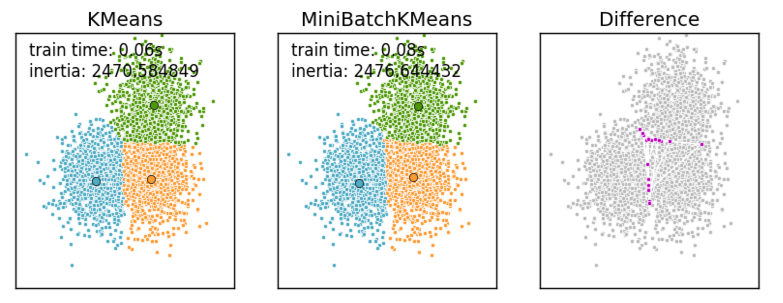6. K-Means与KNN

初学者很容易把K-Means和KNN搞混，两者其实差别还是很大的。

K-Means是无监督学习的聚类算法，没有样本输出；而KNN是监督学习的分类算法，有对应的类别输出。KNN基本不需要训练，对测试集里面的点，只需要找到在训练集中最近的k个点，用这最近的k个点的类别来决定测试点的类别。而K-Means则有明显的训练过程，找到k个类别的最佳质心，从而决定样本的簇类别。

当然，两者也有一些相似点，两个算法都包含一个过程，即找出和某一个点最近的点。两者都利用了最近邻(nearest neighbors)的思想。

列个表格对比一下特点：

KNN

K-Means

1.KNN是分类算法

2.监督学习

3.喂给它的数据集是带label的数据，已经是完全正确的数据

1.K-Means是聚类算法

2.非监督学习

3.喂给它的数据集是无label的数据，是杂乱无章的，经过聚类后才变得有点顺序，先无序，后有序

没有明显的前期训练过程，属于memory-based learning
有明显的前期训练过程
K的含义：来了一个样本x，要给它分类，即求出它的y，就从数据集中，在x附近找离它最近的K个数据点，这K个数据点，类别c占的个数最多，就把x的label设为c
K的含义：K是人工固定好的数字，假设数据集合可以分为K个簇，由于是依靠人工定好，需要一点先验知识

相似点：都包含这样的过程，给定一个点，在数据集中找离它最近的点。即二者都用到了NN(Nears Neighbor)算法，一般用KD树来实现NN。

7.应用实例

这是官网的一个例子，详细参见：http://scikit-learn.org/stable/auto_examples/cluster/plot_color_quantization.html#sphx-glr-auto-examples-cluster-plot-color-quantization-py

执行夏宫（中国）图像的逐像素矢量量化（VQ），将显示图像所需的颜色数量从96,615种独特颜色减少到64，同时保持整体外观质量。

在该示例中，像素在3D空间中表示，并且K均值用于找到64个颜色簇。在图像处理文献中，从K-means（聚类中心）获得的码本被称为调色板。使用单个字节，可以寻址多达256种颜色，而RGB编码每个像素需要3个字节。例如，GIF文件格式使用这样的调色板。

为了比较，还示出了使用随机码本（随机拾取的颜色）的量化图像。

print(__doc__)
import numpy as np
import matplotlib.pyplot as plt
from sklearn.cluster import KMeans
from sklearn.metrics import pairwise_distances_argmin
from sklearn.utils import shuffle
from time import time

n_colors = 64

# Load the Summer Palace photo

# Convert to floats instead of the default 8 bits integer coding. Dividing by
# 255 is important so that plt.imshow behaves works well on float data (need to
# be in the range [0-1])
china = np.array(china, dtype=np.float64) / 255

# Load Image and transform to a 2D numpy array.
w, h, d = original_shape = tuple(china.shape)
assert d == 3
image_array = np.reshape(china, (w * h, d))

print("Fitting model on a small sub-sample of the data")
t0 = time()
image_array_sample = shuffle(image_array, random_state=0)[:1000]
kmeans = KMeans(n_clusters=n_colors, random_state=0).fit(image_array_sample)
print("done in %0.3fs." % (time() - t0))

# Get labels for all points
print("Predicting color indices on the full image (k-means)")
t0 = time()
labels = kmeans.predict(image_array)
print("done in %0.3fs." % (time() - t0))

codebook_random = shuffle(image_array, random_state=0)[:n_colors + 1]
print("Predicting color indices on the full image (random)")
t0 = time()
labels_random = pairwise_distances_argmin(codebook_random,
image_array,
axis=0)
print("done in %0.3fs." % (time() - t0))

def recreate_image(codebook, labels, w, h):
"""Recreate the (compressed) image from the code book & labels"""
d = codebook.shape
image = np.zeros((w, h, d))
label_idx = 0
for i in range(w):
for j in range(h):
image[i][j] = codebook[labels[label_idx]]
label_idx += 1
return image

# Display all results, alongside original image
plt.figure(1)
plt.clf()
ax = plt.axes([0, 0, 1, 1])
plt.axis('off')
plt.title('Original image (96,615 colors)')
plt.imshow(china)

plt.figure(2)
plt.clf()
ax = plt.axes([0, 0, 1, 1])
plt.axis('off')
plt.title('Quantized image (64 colors, K-Means)')
plt.imshow(recreate_image(kmeans.cluster_centers_, labels, w, h))

plt.figure(3)
plt.clf()
ax = plt.axes([0, 0, 1, 1])
plt.axis('off')
plt.title('Quantized image (64 colors, Random)')
plt.imshow(recreate_image(codebook_random, labels_random, w, h))
plt.show()

第二个例子：

实例说明：利用sklearn.datasets.make_blobs产生1500条两维的数据集进行不同情况下的聚类示例，参见网址：https://blog.csdn.net/gamer_gyt/article/details/51244850

代码如下

import numpy as np      #科学计算包
import matplotlib.pyplot as plt      #python画图包

from sklearn.cluster import KMeans       #导入K-means算法包
from sklearn.datasets import make_blobs

plt.figure(figsize=(12, 12))

"""
make_blobs函数是为聚类产生数据集
产生一个数据集和相应的标签
n_samples:表示数据样本点个数,默认值100
n_features:表示数据的维度，默认值是2
centers:产生数据的中心点，默认值3
cluster_std：数据集的标准差，浮点数或者浮点数序列，默认值1.0
center_box：中心确定之后的数据边界，默认值(-10.0, 10.0)
shuffle ：洗乱，默认值是True
random_state:官网解释是随机生成器的种子
更多参数即使请参考：http://scikit-learn.org/dev/modules/generated/sklearn.datasets.make_blobs.html#sklearn.datasets.make_blobs
"""
n_samples = 1500
random_state = 170
X, y = make_blobs(n_samples=n_samples, random_state=random_state)

# Incorrect number of clusters
y_pred = KMeans(n_clusters=2, random_state=random_state).fit_predict(X)

plt.subplot(221)  #在2图里添加子图1
plt.scatter(X[:, 0], X[:, 1], c=y_pred) #scatter绘制散点
plt.title("Incorrect Number of Blobs")   #加标题

# Anisotropicly distributed data
transformation = [[ 0.60834549, -0.63667341], [-0.40887718, 0.85253229]]
X_aniso = np.dot(X, transformation)    #返回的是乘积的形式
y_pred = KMeans(n_clusters=3, random_state=random_state).fit_predict(X_aniso)

plt.subplot(222)#在2图里添加子图2
plt.scatter(X_aniso[:, 0], X_aniso[:, 1], c=y_pred)
plt.title("Anisotropicly Distributed Blobs")

# Different variance
X_varied, y_varied = make_blobs(n_samples=n_samples,
cluster_std=[1.0, 2.5, 0.5],
random_state=random_state)
y_pred = KMeans(n_clusters=3, random_state=random_state).fit_predict(X_varied)

plt.subplot(223)#在2图里添加子图3
plt.scatter(X_varied[:, 0], X_varied[:, 1], c=y_pred)
plt.title("Unequal Variance")

# Unevenly sized blobs
X_filtered = np.vstack((X[y == 0][:500], X[y == 1][:100], X[y == 2][:10]))
y_pred = KMeans(n_clusters=3, random_state=random_state).fit_predict(X_filtered)

plt.subplot(224)#在2图里添加子图4
plt.scatter(X_filtered[:, 0], X_filtered[:, 1], c=y_pred)
plt.title("Unevenly Sized Blobs")

plt.show() #显示图</span>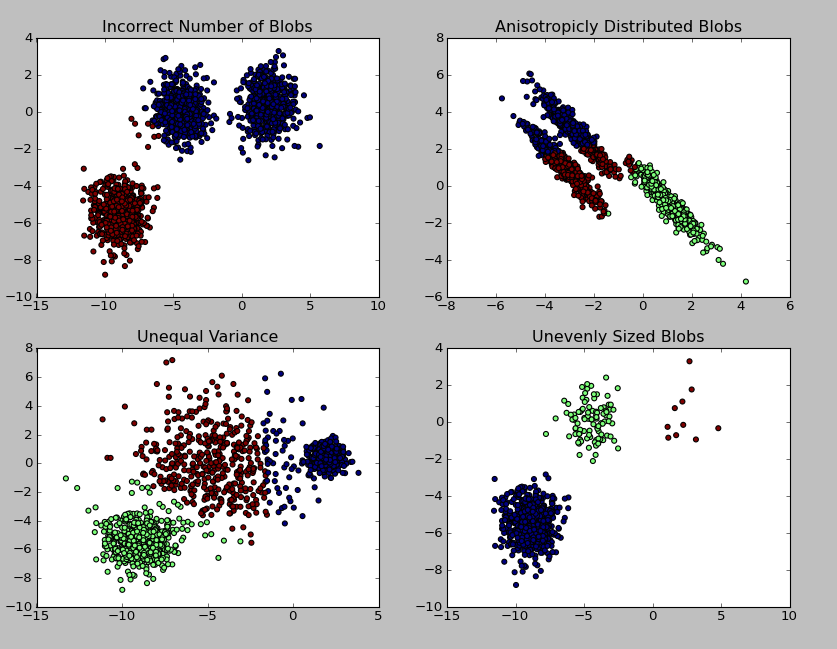参考网址：

https://www.cnblogs.com/pinard/p/6164214.html

https://blog.csdn.net/sinat_35512245/article/details/55051306

https://en.wikipedia.org/wiki/K-means_clustering

http://scikit-learn.org/stable/auto_examples/cluster/plot_color_quantization.html#sphx-glr-auto-examples-cluster-plot-color-quantization-py


展开全文K-means
• ## K-Means（聚类）

万次阅读 多人点赞 2020-07-14 10:42:57
说到聚类，应先理解聚类和分类的区别，很多业务人员在日常分析时候不是很严谨，混为一谈，其实二者有本质的区别。 分类：分类其实是从特定的数据中挖掘模式，作出判断的过程。比如Gmail邮箱里有垃圾邮件分类器，...
说到聚类，应先理解聚类和分类的区别，很多业务人员在日常分析时候不是很严谨，混为一谈，其实二者有本质的区别。

分类：分类其实是从特定的数据中挖掘模式，作出判断的过程。比如Gmail邮箱里有垃圾邮件分类器，一开始的时候可能什么都不过滤，在日常使用过程中，我人工对于每一封邮件点选“垃圾”或“不是垃圾”，过一段时间，Gmail就体现出一定的智能，能够自动过滤掉一些垃圾邮件了。这是因为在点选的过程中，其实是给每一条邮件打了一个“标签”，这个标签只有两个值，要么是“垃圾”，要么“不是垃圾”，Gmail就会不断研究哪些特点的邮件是垃圾，哪些特点的不是垃圾，形成一些判别的模式，这样当一封信的邮件到来，就可以自动把邮件分到“垃圾”和“不是垃圾”这两个我们人工设定的分类的其中一个。

聚类：聚类的目的也是把数据分类，但是事先我是不知道如何去分的，完全是算法自己来判断各条数据之间的相似性，相似的就放在一起。在聚类的结论出来之前，我完全不知道每一类有什么特点，一定要根据聚类的结果通过人的经验来分析，看看聚成的这一类大概有什么特点。

聚类和分类最大的不同在于：分类的目标是事先已知的，而聚类则不一样，聚类事先不知道目标变量是什么，类别没有像分类那样被预先定义出来。

K-Means

聚类算法有很多种（几十种），K-Means是聚类算法中的最常用的一种，算法最大的特点是简单，好理解，运算速度快，但是只能应用于连续型的数据，并且一定要在聚类前需要手工指定要分成几类。

下面，我们描述一下K-means算法的过程，为了尽量不用数学符号，所以描述的不是很严谨，大概就是这个意思，“物以类聚、人以群分”：

首先输入k的值，即我们希望将数据集经过聚类得到k个分组。
从数据集中随机选择k个数据点作为初始大哥（质心，Centroid）
对集合中每一个小弟，计算与每一个大哥的距离（距离的含义后面会讲），离哪个大哥距离近，就跟定哪个大哥。
这时每一个大哥手下都聚集了一票小弟，这时候召开人民代表大会，每一群选出新的大哥（其实是通过算法选出新的质心）。
如果新大哥和老大哥之间的距离小于某一个设置的阈值（表示重新计算的质心的位置变化不大，趋于稳定，或者说收敛），可以认为我们进行的聚类已经达到期望的结果，算法终止。
如果新大哥和老大哥距离变化很大，需要迭代3~5步骤。

例：

我搞了6个点，从图上看应该分成两推儿，前三个点一堆儿，后三个点是另一堆儿。现在手工执行K-Means，体会一下过程，同时看看结果是不是和预期一致。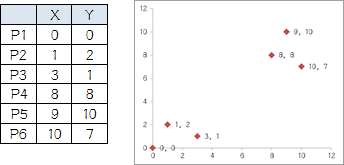case

1.选择初始大哥： 我们就选P1和P2

2.计算小弟和大哥的距离： P3到P1的距离从图上也能看出来（勾股定理），是√10 = 3.16；P3到P2的距离√((3-1)^2+(1-2)^2 = √5 = 2.24，所以P3离P2更近，P3就跟P2混。同理，P4、P5、P6也这么算，如下：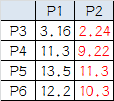round1

P3到P6都跟P2更近，所以第一次站队的结果是：

组A：P1
组B：P2、P3、P4、P5、P6
3.人民代表大会： 组A没啥可选的，大哥还是P1自己 组B有五个人，需要选新大哥，这里要注意选大哥的方法是每个人X坐标的平均值和Y坐标的平均值组成的新的点，为新大哥，也就是说这个大哥是“虚拟的”。 因此，B组选出新大哥的坐标为：P哥（（1+3+8+9+10）/5，（2+1+8+10+7）/5）=（6.2，5.6）。 综合两组，新大哥为P1（0，0），P哥（6.2，5.6），而P2-P6重新成为小弟

4.再次计算小弟到大哥的距离：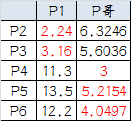round2

这时可以看到P2、P3离P1更近，P4、P5、P6离P哥更近，所以第二次站队的结果是：

组A：P1、P2、P3
组B：P4、P5、P6（虚拟大哥这时候消失）
5.第二届人民代表大会： 按照上一届大会的方法选出两个新的虚拟大哥：P哥1（1.33，1） P哥2（9，8.33），P1-P6都成为小弟

6.第三次计算小弟到大哥的距离：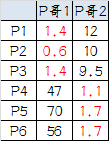round3

这时可以看到P1、P2、P3离P哥1更近，P4、P5、P6离P哥2更近，所以第二次站队的结果是：

组A：P1、P2、P3
组B：P4、P5、P6
我们发现，这次站队的结果和上次没有任何变化了，说明已经收敛，聚类结束，聚类结果和我们最开始设想的结果完全一致。

K-Means的细节问题：

K值怎么定？我怎么知道应该几类？ 答：这个真的没有确定的做法，分几类主要取决于个人的经验与感觉，通常的做法是多尝试几个K值，看分成几类的结果更好解释，更符合分析目的等。或者可以把各种K值算出的SSE做比较，取最小的SSE的K值。

初始的K个质心怎么选？ 答：最常用的方法是随机选，初始质心的选取对最终聚类结果有影响，因此算法一定要多执行几次，哪个结果更reasonable，就用哪个结果。 当然也有一些优化的方法，第一种是选择彼此距离最远的点，具体来说就是先选第一个点，然后选离第一个点最远的当第二个点，然后选第三个点，第三个点到第一、第二两点的距离之和最小，以此类推。第二种是先根据其他聚类算法（如层次聚类）得到聚类结果，从结果中每个分类选一个点。

K-Means会不会陷入一直选质心的过程，永远停不下来？ 答：不会，有数学证明K-Means一定会收敛，大致思路是利用SSE的概念（也就是误差平方和），即每个点到自身所归属质心的距离的平方和，这个平方和是一个函数，然后能够证明这个函数是可以最终收敛的函数。

判断每个点归属哪个质心的距离怎么算？ 答：这个问题必须不得不提一下数学了…… 第一种，欧几里德距离（欧几里德这位爷还是很厉害的，《几何原本》被称为古希腊数学的高峰，就是用5个公理推导出了整个平面几何的结论），这个距离就是平时我们理解的距离，如果是两个平面上的点，也就是（X1，Y1），和（X2，Y2），那这俩点距离是多少初中生都会，就是√( (x1-x2)^2+(y1-y2)^2） ，如果是三维空间中呢？√( (x1-x2)^2+(y1-y2)^2+(z1-z2)^2 ；推广到高维空间公式就以此类推。可以看出，欧几里德距离真的是数学加减乘除算出来的距离，因此这就是只能用于连续型变量的原因。 第二种，余弦相似度，余弦相似度用向量空间中两个向量夹角的余弦值作为衡量两个个体间差异的大小。相比距离度量，余弦相似度更加注重两个向量在方向上的差异，而非距离或长度上。下图表示余弦相似度的余弦是哪个角的余弦，A，B是三维空间中的两个向量，这两个点与三维空间原点连线形成的角，如果角度越小，说明这两个向量在方向上越接近，在聚类时就归成一类：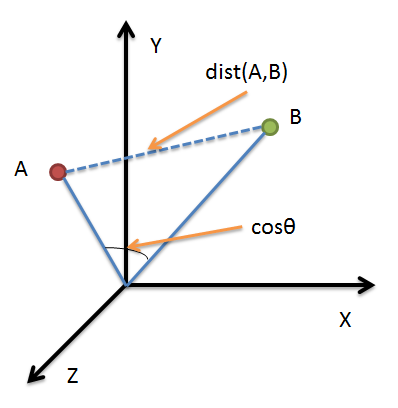cos

看一个例子（也许不太恰当）：歌手大赛，三个评委给三个歌手打分，第一个评委的打分（10，8，9） 第二个评委的打分（4，3，2），第三个评委的打分（8，9，10） 如果采用余弦相似度来看每个评委的差异，虽然每个评委对同一个选手的评分不一样，但第一、第二两个评委对这四位歌手实力的排序是一样的，只是第二个评委对满分有更高的评判标准，说明第一、第二个评委对音乐的品味上是一致的。 因此，用余弦相似度来看，第一、第二个评委为一类人，第三个评委为另外一类。 如果采用欧氏距离， 第一和第三个评委的欧氏距离更近，就分成一类人了，但其实不太合理，因为他们对于四位选手的排名都是完全颠倒的。 总之，如果注重数值本身的差异，就应该用欧氏距离，如果注重的是上例中的这种的差异（我概括不出来到底是一种什么差异……），就要用余弦相似度来计算。 还有其他的一些计算距离的方法，但是都是欧氏距离和余弦相似度的衍生，简单罗列如下：明可夫斯基距离、切比雪夫距离、曼哈顿距离、马哈拉诺比斯距离、调整后的余弦相似度、Jaccard相似系数……

还有一个重要的问题是，大家的单位要一致！ 比如X的单位是米，Y也是米，那么距离算出来的单位还是米，是有意义的 但是如果X是米，Y是吨，用距离公式计算就会出现“米的平方”加上“吨的平方”再开平方，最后算出的东西没有数学意义，这就有问题了。 还有，即使X和Y单位一致，但是如果数据中X整体都比较小，比如都是1到10之间的数，Y很大，比如都是1000以上的数，那么，在计算距离的时候Y起到的作用就比X大很多，X对于距离的影响几乎可以忽略，这也有问题。 因此，如果K-Means聚类中选择欧几里德距离计算距离，数据集又出现了上面所述的情况，就一定要进行数据的标准化（normalization），即将数据按比例缩放，使之落入一个小的特定区间。去除数据的单位限制，将其转化为无量纲的纯数值，便于不同单位或量级的指标能够进行计算和比较。 标准化方法最常用的有两种：

min-max标准化（离差标准化）：对原始数据进行线性变换，是结果落到【0，1】区间，转换方法为 X'=（X-min）/（max-min），其中max为样本数据最大值，min为样本数据最小值。
z-score标准化（标准差标准化）：处理后的数据符合标准正态分布（均值为0，方差为1），转换公式：X减去均值，再除以标准差

每一轮迭代如何选出新的质心？ 答：各个维度的算术平均，比如（X1，Y1，Z1）、（X2，Y2，Z2）、（X3，Y3，Z3），那就新质心就是【（X1+X2+X3）/3，（Y1+Y2+Y3）/3，（Z1，Z2，Z3）/3】，这里要注意，新质心不一定是实际的一个数据点。

关于离群值？ 答：离群值就是远离整体的，非常异常、非常特殊的数据点，在聚类之前应该将这些“极大”“极小”之类的离群数据都去掉，否则会对于聚类的结果有影响。但是，离群值往往自身就很有分析的价值，可以把离群值单独作为一类来分析。

用SPSS作出的K-Means聚类结果，包含ANOVA（单因素方差分析)，是什么意思？ 答：答简单说就是判断用于聚类的变量是否对于聚类结果有贡献，方差分析检验结果越显著的变量，说明对聚类结果越有影响。对于不显著的变量，可以考虑从模型中剔除。

聚类分析中业务专家的作用：

业务专家的作用非常大，主要体现在聚类变量的选择和对于聚类结果的解读：

比如要对于现有的客户分群，那么就要根据最终分群的目的选择不同的变量来分群，这就需要业务专家经验支持。如果要优化客户服务的渠道，那么就应选择与渠道相关的数据；如果要推广一个新产品，那就应该选用用户目前的使用行为的数据来归类用户的兴趣。算法是无法做到这一点的
欠缺经验的分析人员和经验丰富的分析人员对于结果的解读会有很大差异。其实不光是聚类分析，所有的分析都不能仅仅依赖统计学家或者数据工程师。
转载：https://www.jianshu.com/p/fc91fed8c77b

展开全文K-Means 聚类
• ## K-means方法总结（附代码）

千次阅读 热门讨论 2019-04-26 16:50:03
K-means方法总结（附代码） 这一周事情较多，不得已先放弃了验证码分割部分的卷积神经网络的学习，先写两篇关于聚类方法的内容，分别是k-means和混合高斯模型。因为之前的论文中有关于k-means方法的字符分割方法，...k-means 聚类
• 用动画效果 帮助新手理解K-means聚类，有数据，有动画数据挖掘
• 一个是用来将数据划分到不同类别的 k-means 算法，一个是用来提取重要特征并给特征降维的 PCA 算法。 点击 课程视频 你就能不间断地学习 Ng 的课程，关于课程作业的 Python 代码已经放到了 Github 上，点击 课程...吴恩达 无监督学习 聚类 k-means PCA
• 本压缩包包括了K-SVD，k-means的python代码实现，同时还提供了自制的PPT详解，同时包括K-SVD最经典的论文一篇，内容充实易懂，欢迎大家学习下载。
• ## K-means & K-SVD原理

千次阅读 2019-02-27 20:47:33
K-means算法多用于聚类 K-SVD算法则可用于压缩，编码，聚类等功能 稀疏表示 用较少的基本信号的线性组合来表达大部分或者全部的原始信号。 每个矩阵的列向量可看成一个信号，一个矩阵则是信号的集合。 ...机器学习 聚类
• K-MeansK-MeansK-Means算法K-Means缺点:K-Means优化K-Means实现 K-Means K-Means是最为经典的无监督聚类（Unsupervised Clustering）算法，其主要目的是将n个样本点划分为k个簇，使得相似的样本尽量被分到同一个聚簇...聚类 K-Means nlp
• ## 机器学习之k-means算法详解

万次阅读 多人点赞 2019-04-15 14:03:35
K-means算法 （无监督算法，聚类算法） 1-1 基本流程 一、概念： 二、主要特点： 三、算法流程： kmeans作用：去除奇异值 小结： 1-2 算法效果衡量标准 一、K值确定： 二、轮廓系数： 三、Canopy算法配合初始...kmeans
• ## 深入理解K-Means聚类算法

万次阅读 多人点赞 2016-12-18 20:50:05
概述什么是聚类分析聚类分析是在数据中发现数据对象之间的关系，将数据进行分组，组内的相似性越大，组间的差别越大，则聚类效果越好。不同的簇类型聚类旨在发现有用的对象簇，在现实中我们用到很多的簇的类型，使用...k-means 聚类算法
• ML之Clustering之K-meansK-means算法简介、应用、经典案例之详细攻略 目录 K-means算法简介 1、K-means算法适用的数据类型​ 2、K-Means算法的全局最优解和局部最优解的比较 1、K-means算法的过程及其...
• 代码：https://github.com/dengsiying/K-Means-improvement.gitK-Means聚类算法的研究与改进*1(华中师范大学 计算机学院,湖北 武汉 430079)摘 要:K-Means算法是基于划分的聚类算法中的一个典型算法,该算法有操作...
• ## K-Means优缺点

万次阅读 2018-07-12 00:10:33
k-means的优点有：1.原理简单，实现方便，收敛速度快；2.聚类效果较优；3.模型的可解释性较强；4.调参只需要簇数kk-means的缺点有：1.k的选取不好把握；2.对于不是凸的数据集比较难以收敛；3.如果数据的类型不平衡...
• ## K-means算法研究综述

万次阅读 2017-03-18 10:55:01
K-means算法研究综述 聚类被认为是机器学习中最常使用的技术之一, 它历史悠久、应用广泛，几乎应用于环境学、医学、生物学、天文学、经济学等各个领域。其中K-means是最为常用的聚类算法。现在我们来详细介绍一下...机器学习 Kmeans
• ## k-means算法详解

万次阅读 2018-05-03 11:58:19
k-means算法详解 主要内容 k-means算法简介 k-means算法详解 k-means算法优缺点分析 k-means算法改进算法k-means++ 1、k-means算法简介   k-means算法是一种聚类算法，所谓聚类，即根据相似性原则，将具有...算法
• K-Means算法有大量的变体，本文就从最传统的K-Means算法讲起，在其基础上讲述K-Means的优化变体方法。包括初始化优化K-Means++, 距离计算优化elkan K-Means算法和大数据情况下的优化Mini Batch K-Means算法。 1. ...机器学习
• ## k-means算法优缺点

千次阅读 2018-04-10 20:37:20
K-Means的主要优点： 1）原理简单，容易实现 2）可解释度较强 K-Means的主要缺点： 1）K值的选取困难 2）局部最优 3）对噪音和异常点敏感 4）需样本存在均值（限定数据种类） 5）聚类效果依赖于聚类中心的初始化 6...机器学习 算法
• 一、k-means：在大数据的条件下，会耗费大量的时间和内存。 优化k-means的建议： 1、减少聚类的数目K。因为，每个样本都要跟类中心计算距离。 2、减少样本的特征维度。比如说，通过PCA等进行降维。 3、考察其他的...机器学习 k-means
...